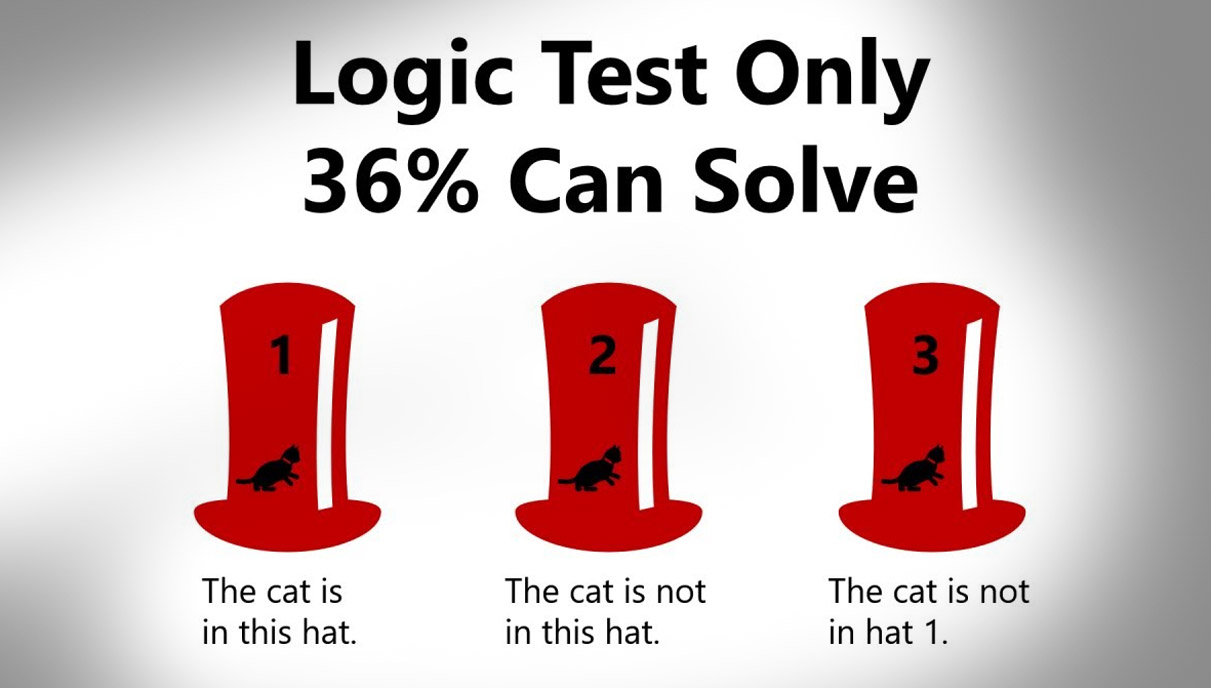# 64 Percent Of People Can’t Solve This Simple ‘Cat In The Hat’ Logic TestAccording to YouTube channel Mind Your Decisions, only 36 percent of people could solve this relatively straightforward “Cat in the Hat” logic problem.

The Shorty Award nominated channel, which boasts almost 2 million subscribers and is run by economics and math expert Presh Talwalkar, posts brain teasers, riddles, logic puzzles, and viral math problems.

This is the latest test.

The Rules: Only one hat contains a cat. Only one of the statements below is true.

Hat 1: the cat is in this hat.
Hat 2: the cat is not in this hat.
Hat 3: the cat is not in hat 1.

(As shown in the photo above.)

The Question: Which hat contains the cat?

a) Hat 1
b) Hat 2
c) Hat 3
d) None of them
e) Not enough information

Have you figured out the answer?

We’ll wait.

No rush.

Related: If You Can Solve This Brain Teaser, The CIA Would Like To Talk To You About A Job

Okay, that’s long enough.

Here’s the answer and the logic behind it.

First, you have to assume the cat is in each hat. Then you need to figure out which of the three statements is actually true, and which two statements are false.

So, if the cat is in Hat 1, then the first two statements are true. The third is not.

Let’s then skip to Hat 3. If the cat is under Hat 3 then the second and third statements are true and the first statement is not.

Now, if the cat is in Hat 2, then the first two statements are false and only the third statement is true.

Remember, the rules of the problem state that only one of the statements is true. So which hat is the cat under?

Confused? I feel you. Watch this…